Updated by Jon Samuelson on Nov 04, 2019REPORTJon Samuelson
Owner
10 items   6 followers   0 votes   3.37k views

# Elementary Math Websites To Practice Place Value + Decimals

A list developed by Cindy Lawrence, 3rd grade teacher in Texas. This list accompanies Unit 5 of the Third Grade Everyday Math Curriculum.

1

## Scooter Quest Place Value Game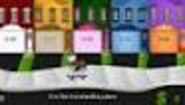Learn place value models with this fun math game that involves shooting the fruit with the correct math answers.

2

## Elementary Math - Decimal Tiles - Virtual ManipulativesABCya! Decimal tiles are a good way for students to explore and understand how parts make up a whole. Teachers and parents can utilize this resource when giving a lesson on decimals with students.

3

## Math Games: Decimals Tenths - Matching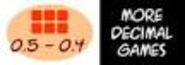Learn decimals the fun way with this decimal model game.

4

## Math Games: Decimals Hundredths - Matching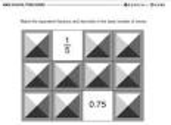5

## Math Games: Fruit Shoot Decimal Comparisons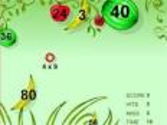Learn how to compare decimals the fun way with Fruit Shoot Decimal Comparisons math game.

6

## Balloon Pop Math - Decimal Patterns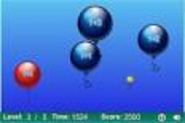Learn about decimal patterns and sequences with this fun math game.

7

## Scooter Quest Compare Decimals to Fractions Math Game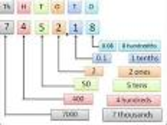Scooter Quest math game. Have fun comparing decimals to fractions.

8

## Fractions, Decimals, Percents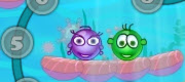Action packed math site for elementary and middle school students featuring math games, math word problems, math worksheets, logic puzzles, and math videos.

9

## BBC - Decimals activityAn animated KS2 activity for the Maths topic: Decimals

10

## BBC - KS2 Maths - Percentages activityAn animated KS2 activity for the Maths topic: Percentages